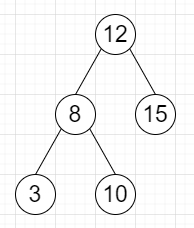# Program to find k-length paths on a binary tree in Python

Suppose we have a binary tree which contains unique values and we also have another value k, we have to find the number of k-length unique paths in the tree. The paths can go either from parent to child or from child to parent. We will consider two paths are different when some node appears in one path but not the other.

So, if the input is likek = 3, then the output will be 4, as the paths are [12,8,3], [12,8,10], [8,12,15], [3,8,10].

To solve this, we will follow these steps−

• Define a function dfs() . This will take node

• if node is null, then

• return a list with 1 and k-1 number of 0s

• left := dfs(left of node)

• right := dfs(right of node)

• for i in range 0 to K, do

• ans := ans + left[i] * right[K - 1 - i]

• res := a list of size K of 0s

• res := 1, res := 1

• for i in range 1 to K - 1, do

• res[i + 1] := res[i + 1] + left[i]

• res[i + 1] := res[i + 1] + right[i]

• return res

• From the main method, do the following−

• ans := 0

• dfs(root)

• return ans

Let us see the following implementation to get better understanding −

## Example

Live Demo

class TreeNode:
def __init__(self, data, left = None, right = None):
self.data = data
self.left = left
self.right = right
class Solution:
def solve(self, root, K):
def dfs(node):
if not node:
return  +  * (K-1)
left = dfs(node.left)
right = dfs(node.right)
for i in range(K):
self.ans += left[i] * right[K - 1 - i]
res =  * K
res = res = 1
for i in range(1, K - 1):
res[i + 1] += left[i]
res[i + 1] += right[i]
return res
self.ans = 0
dfs(root)
return self.ans
ob = Solution()
root = TreeNode(12)
root.left = TreeNode(8)
root.right = TreeNode(15)
root.left.left = TreeNode(3)
root.left.right = TreeNode(10)
print(ob.solve(root, 3))

## Input

root = TreeNode(12)
root.left = TreeNode(8)
root.right = TreeNode(15)
root.left.left = TreeNode(3)
root.left.right = TreeNode(10)
3

## Output

4